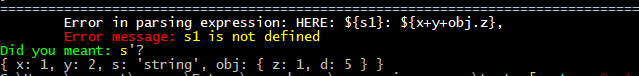Nordic Panda Moor

# npm

## @nexssp/expression-parser

1.0.3 • Public • Published

# @nexssp/expression-parser

Easy Object expression-parser. See below for details.

NOTE: This module is experimental! It works, but may have some issues. Submit new issue if you have found any issue..

## Installation

`npm i @nexssp/expression-parser`

## Usage

### When there is error in parsing

It shows the error with suggestion when there is type for example:## Expression Parser

```const ep = require("@nexssp/expression-parser");

const data = { x: 1, y: 2, s: "some string", obj: { z: 1, d: 5 } };
console.log(ep.expressionParser("HERE: \${s}: \${x+y+obj.z}", data));

// Result: HERE: some string: 4```

### parseData

```const ep = require("@nexssp/expression-parser");

const data = {
my1stVar: "myfirst variable",
number: 5,
another: 10,
functionx: (y) => {
x = 3;
return x + y;
},
something: "Here is evaluated the object itself: \${my1stVar}",
calculate: "Some calculations working: \${number+another} \${functionx(1000)}",
ommitedField: "Will not be evaluated: \${number+another}",
x: "\${a+b+C}", // Will not show error if a, b or C does not exist. as it is not evaluated.
};

console.log(ep.parseData(data, ["ommitedField", "x"]));```

Result below. You can even use functions defined in the object etc. see \${functionx(1000)}

```{
my1stVar: 'myfirst variable',
number: 5,
another: 10,
functionx: [Function: functionx],
something: 'Here is evaluated the object itself: myfirst variable',
calculate: 'Some calculations working: 15 1003',
ommitedField: 'Will not be evaluated: \${number+another}',
x: '\${a+b+C}'
}```

## Keywords

### Install

`npm i @nexssp/expression-parser`

### Repository

github.com/nexssp/test

nexss.com

14

1.0.3